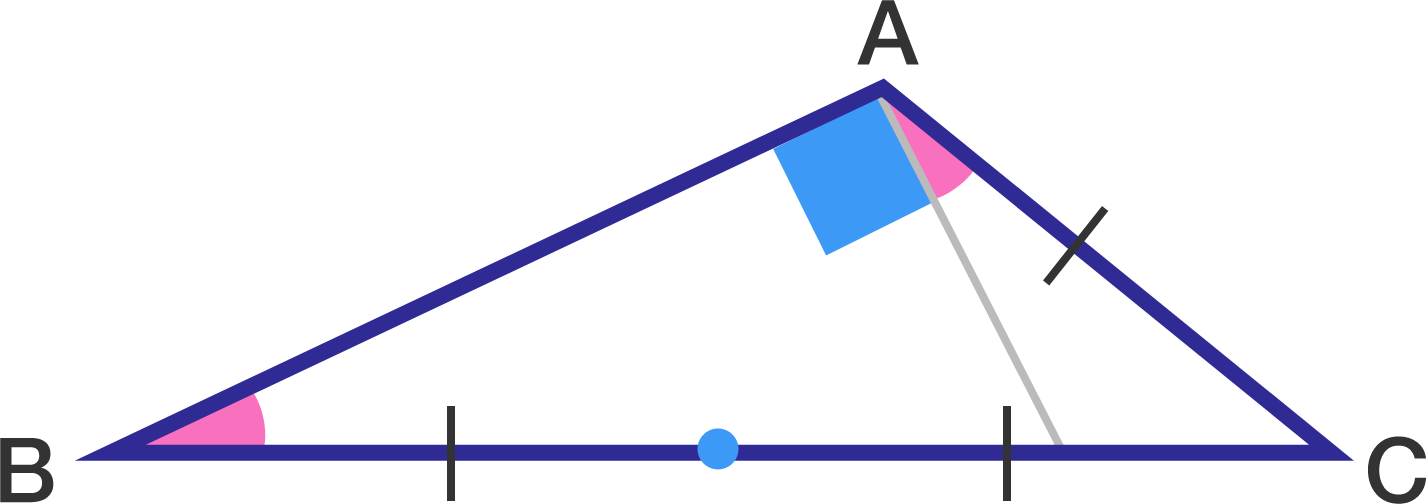# Trigonometry!

Geometry Level 2

In $\Delta ABC$, if the length of $BC$ is twice the length of $AC,$ and $\angle A-\angle B=90^\circ,$ what is the value of $\tan C$?×